• 该篇博文是对librosa能量_librosa语音信号处理重新编辑 读取音频 读取音频文件。默认采样率是22050，如果要保留音频的原始采样率，使用sr = None。 y, sr = librosa.load...# offset：float，在此时间之后开始阅读(以
该篇博文是对librosa能量_librosa语音信号处理重新编辑
读取音频
读取音频文件。默认采样率是22050，如果要保留音频的原始采样率，使用sr = None。
y, sr = librosa.load(path, sr=22050, mono=True, offset=0.0, duration=None)
# path：音频文件的路径。
# sr：采样率，如果为“None”使用音频自身的采样率
# mono：bool，是否将信号转换为单声道
# offset：float，在此时间之后开始阅读(以秒为单位)
# duration：float，持续时间,仅加载这么多的音频(以秒为单位)
# y：音频时间序列
# sr：音频的采样率


重采样
重新采样从orig_sr到target_sr的时间序列
y_hat = librosa.resample(y, orig_sr, target_sr, fix=True, scale=False)
# y：音频时间序列。可以是单声道或立体声。
# orig_sr：y的原始采样率
# target_sr：目标采样率
# fix：bool，调整重采样信号的长度，使其大小恰好为 $\frac{len(y)}{orig\_sr}*target\_sr =t*target\_sr$
# scale：bool，缩放重新采样的信号，以使y和y_hat具有大约相等的总能量。
# y_hat：重采样之后的音频数组

读取时长
计算时间序列的的持续时间(以秒为单位)
d = librosa.get_duration(y=None, sr=22050, S=None, n_fft=2048, hop_length=512, center=True, filename=None)
# y：音频时间序列
# sr：y的音频采样率
# S：STFT矩阵或任何STFT衍生的矩阵(例如，色谱图或梅尔频谱图)。根据频谱图输入计算的持续时间仅在达到帧分辨率之前才是准确的。如果需要高精度，则最好直接使用音频时间序列。
# n_fft：S的 FFT窗口大小
# hop_length：S列之间的音频样本数
# center ：布尔值 如果为True，则S [:, t]的中心为y [t * hop_length]如果为False，则S [:, t]从y[t * hop_length]开始
# filename：如果提供，则所有其他参数都将被忽略，并且持续时间是直接从音频文件中计算得出的。
# d：持续时间(以秒为单位)

读取采样率
sr = librosa.get_samplerate(path)

写音频
将时间序列输出为.wav文件
librosa.output.write_wav(path, y, sr, norm=False)
# path：保存输出wav文件的路径
# y：音频时间序列。
# sr：y的采样率
# norm：bool，是否启用幅度归一化。将数据缩放到[-1，+1]范围。

在0.8.0以后的版本，librosa都会将这个函数删除，推荐用以下函数soundfile.write：
importsoundfile
soundfile.write(file, data, samplerate)
# file：保存输出wav文件的路径
# data：音频数据
# samplerate：采样率

过零率
计算音频时间序列的过零率。
zcr = librosa.feature.zero_crossing_rate(y, frame_length = 2048, hop_length = 512, center = True)
# y：音频时间序列
# frame_length：帧长
# hop_length：帧移
# center：bool，如果为True，则通过填充y的边缘来使帧居中。
# zcr：zcr[0，i]是第i帧中的过零率

应用：
y, sr =librosa.load(librosa.util.example_audio_file())
print(librosa.feature.zero_crossing_rate(y))

#array([[ 0.134, 0.139, ..., 0.387, 0.322]])

波形图
绘制波形的幅度包络线
librosa.display.waveplot(y, sr=22050, x_axis='time', offset=0.0, ax=None)
# y：音频时间序列
# sr：y的采样率
# x_axis：str {'time'，'off'，'none'}或None，如果为“时间”，则在x轴上给定时间刻度线。
# offset：水平偏移(以秒为单位)开始波形图

应用：
importlibrosa.displayimportmatplotlib.pyplot as plt
librosa.display.waveplot(y, sr=sr)
plt.show()

短时傅里叶变换
短时傅立叶变换(STFT)，返回一个复数矩阵使得D(f,t)，复数的实部：np.abs(D(f,t))频率的振幅；复数的虚部：np.angle(D(f,t))频率的相位
STFT = librosa.stft(y, n_fft=2048, hop_length=None, win_length=None, window='hann', center=True, dtype=np.complex64, pad_mode='reflect')
# y：音频时间序列
# n_fft：FFT窗口大小，n_fft=hop_length+overlapping
# hop_length：帧移，如果未指定，则默认win_length / 4。
# win_length：每一帧音频都由window()加窗。窗长win_length，然后用零填充以匹配N_FFT。默认win_length=n_fft。
# window：字符串，元组，数字，函数 shape =(n_fft, )窗口(字符串，元组或数字)；窗函数，例如scipy.signal.hanning，长度为n_fft的向量或数组
# center：bool 如果为True，则填充信号y，以使帧 D [:, t]以y [t * hop_length]为中心。如果为False，则D [:, t]从y [t * op_length]开始
# dtype：D的复数值类型。默认值为64-bit complex复数
# STFT矩阵，shape =(1 + $\frac{n_{fft} }{2}$，t)

短时傅里叶逆变换
短时傅立叶逆变换(ISTFT)，将复数值D(f,t)频谱矩阵转换为时间序列y，窗函数、帧移等参数应与stft相同
y = librosa.istft(stft_matrix, hop_length=None, win_length=None, window='hann', center=True, length=None)
# stft_matrix：经过STFT之后的矩阵
# hop_length：帧移，默认为$\frac{win_{length}}{4}$
# win_length：窗长，默认为n_fft
# window：字符串，元组，数字，函数或shape = (n_fft, )窗口(字符串，元组或数字)窗函数，例如scipy.signal.hanning长度为n_fft的向量或数组
# center：bool如果为True，则假定D具有居中的帧如果False，则假定D具有左对齐的帧
# length：如果提供，则输出y为零填充或剪裁为精确长度音频
# y：时域信号

幅度转dB
将幅度频谱转换为dB标度频谱。也就是对S取对数。与这个函数相反的是librosa.db_to_amplitude(S)
S1 = librosa.amplitude_to_db(S, ref=1.0)
# S：输入幅度
# ref：参考值，振幅abs(S)相对于ref进行缩放，$20*log_{10}(\frac{S}{ref})$
# S1: 单位dB

功率转dB
将功率谱(幅度平方)转换为分贝(dB)单位，与这个函数相反的是librosa.db_to_power(S)
S1 = librosa.core.power_to_db(S, ref=1.0)
# S：输入功率
# ref：参考值，振幅abs(S)相对于ref进行缩放，$10*log_{10}(\frac{S}{ref})$
# S1: dB为单位的S

应用
importlibrosa.displayimportnumpy as npimportmatplotlib.pyplot as plt
S=np.abs(librosa.stft(y))print(librosa.power_to_db(S ** 2))#array([[-33.293, -27.32 , ..., -33.293, -33.293],#[-33.293, -25.723, ..., -33.293, -33.293],#...,#[-33.293, -33.293, ..., -33.293, -33.293],#[-33.293, -33.293, ..., -33.293, -33.293]], dtype=float32)
plt.figure()
plt.subplot(2, 1, 1)
librosa.display.specshow(S** 2, sr=sr, y_axis='log') #从波形获取功率谱图
plt.colorbar()
plt.title('Power spectrogram')
plt.subplot(2, 1, 2)#相对于峰值功率计算dB, 那么其他的dB都是负的，注意看后边cmp值
librosa.display.specshow(librosa.power_to_db(S ** 2, ref=np.max),
sr=sr, y_axis='log', x_axis='time')
plt.colorbar(format='%+2.0f dB')
plt.title('Log-Power spectrogram')
plt.set_cmap("autumn")
plt.tight_layout()
plt.show()


频谱图
所有频率类型均以Hz为单位绘制
librosa.display.specshow(data, x_axis=None, y_axis=None, sr=22050, hop_length=512)
# data：要显示的矩阵
# sr：采样率
# hop_length：帧移
# x_axis 、y_axis：x和y轴的范围
# 频率类型
# 'linear'，'fft'，'hz'：频率范围由FFT窗口和采样率确定
# 'log'：频谱以对数刻度显示
# 'mel'：频率由mel标度决定
# 时间类型
# time：标记以毫秒，秒，分钟或小时显示。值以秒为单位绘制。
# s：标记显示为秒。
# ms：标记以毫秒为单位显示。

应用
importlibrosa.displayimportnumpy as npimportmatplotlib.pyplot as plt
plt.figure()
D= librosa.amplitude_to_db(np.abs(librosa.stft(y)), ref=np.max)
plt.subplot(2, 1, 1)
librosa.display.specshow(D, y_axis='linear')
plt.colorbar(format='%+2.0f dB')
plt.title('线性频率功率谱')
plt.subplot(2, 1, 2)
librosa.display.specshow(D, y_axis='log')
plt.colorbar(format='%+2.0f dB')
plt.title('对数频率功率谱')
plt.show()

Mel滤波器组
创建一个滤波器组矩阵以将FFT合并成Mel频率
melfb = librosa.filters.mel(sr, n_fft, n_mels=128, fmin=0.0, fmax=None, htk=False, norm=1)
# sr：输入信号的采样率
# n_fft：FFT组件数
# n_mels：产生的梅尔带数
# fmin：最低频率(Hz)
# fmax：最高频率(以Hz为单位)。如果为None，则使用fmax = sr / 2.0
# norm：{None，1，np.inf} [标量]如果为1，则将三角mel权重除以mel带的宽度(区域归一化)。否则，保留所有三角形的峰值为1.0
# melfb :Mel变换矩阵

应用
melfb = librosa.filters.mel(22050, 2048)#array([[ 0. , 0.016, ..., 0. , 0. ],#[ 0. , 0. , ..., 0. , 0. ],#...,#[ 0. , 0. , ..., 0. , 0. ],#[ 0. , 0. , ..., 0. , 0. ]])
importmatplotlib.pyplot as plt
plt.figure()
librosa.display.specshow(melfb, x_axis='linear')
plt.ylabel('Mel filter')
plt.title('Mel filter bank')
plt.colorbar()
plt.tight_layout()
plt.show()

计算Mel scaled 频谱
如果提供了频谱图输入S，则通过mel_f.dot(S)将其直接映射到mel_f上。如果提供了时间序列输入y，sr，则首先计算其幅值频谱S，然后通过mel_f.dot(S ** power)将其映射到mel scale上 。默认情况下，power= 2在功率谱上运行。
tt = librosa.feature.melspectrogram(y=None, sr=22050, S=None, n_fft=2048, hop_length=512, win_length=None, window='hann',center=True, pad_mode='reflect', power=2.0)
# y：音频时间序列
# sr：采样率
# S：频谱
# n_fft：FFT窗口的长度
# hop_length：帧移
# win_length：窗口的长度为win_length，默认win_length = n_fft
# window：字符串，元组，数字，函数或shape =(n_fft, )窗口函数，例如 scipy.signal.hanning长度为n_fft的向量或数组
# center：bool 如果为True，则填充信号y，以使帧 t以y [t * hop_length]为中心。如果为False，则帧t从y [t * hop_length]开始
# power：幅度谱的指数。例如1代表能量，2代表功率，等等
# n_mels：滤波器组的个数 1288
# fmax：最高频率
# tt: Mel频谱shape=(n_mels, t)

方法一
importlibrosa.displayimportnumpy as npimportmatplotlib.pyplot as plt
print(librosa.feature.melspectrogram(y=y, sr=sr))#array([[ 2.891e-07, 2.548e-03, ..., 8.116e-09, 5.633e-09],#[ 1.986e-07, 1.162e-02, ..., 9.332e-08, 6.716e-09],#...,#[ 3.668e-09, 2.029e-08, ..., 3.208e-09, 2.864e-09],#[ 2.561e-10, 2.096e-09, ..., 7.543e-10, 6.101e-10]])


方法二：使用stft频谱求Mel频谱
D = np.abs(librosa.stft(y)) ** 2 #stft频谱
S = librosa.feature.melspectrogram(S=D) #使用stft频谱求Mel频谱
plt.figure(figsize=(10, 4))
librosa.display.specshow(librosa.power_to_db(S, ref=np.max),
y_axis='mel', fmax=8000, x_axis='time')
plt.colorbar(format='%+2.0f dB')
plt.title('Mel spectrogram')
plt.tight_layout()
plt.show()

提取Log-Mel Spectrogram 特征
Log-Mel Spectrogram特征是目前在语音识别和环境声音识别中很常用的一个特征，由于CNN在处理图像上展现了强大的能力，使得音频信号的频谱图特征的使用愈加广泛，甚至比MFCC使用的更多。在librosa中，Log-Mel Spectrogram特征的提取只需几行代码：
importlibrosa
y, sr= librosa.load('./train_nb.wav', sr=16000)#提取 mel spectrogram feature
melspec = librosa.feature.melspectrogram(y, sr, n_fft=1024, hop_length=512, n_mels=128)
logmelspec= librosa.amplitude_to_db(melspec) #转换到对数刻度
print(logmelspec.shape) #(128, 65)

可见，Log-Mel Spectrogram特征是二维数组的形式，128表示Mel频率的维度(频域)，64为时间帧长度(时域)，所以Log-Mel Spectrogram特征是音频信号的时频表示特征。其中，n_fft指的是窗的大小，这里为1024；hop_length表示相邻窗之间的距离，这里为512，也就是相邻窗之间有50%的overlap；n_mels为mel bands的数量，这里设为128。
提取MFCC系数
MFCC特征是一种在自动语音识别和说话人识别中广泛使用的特征。关于MFCC特征的详细信息，有兴趣的可以参考博客http:// blog.csdn.net/zzc15806/article/details/79246716。在librosa中，提取MFCC特征只需要一个函数：
mfccs  = librosa.feature.mfcc(y=None, sr=22050, S=None, n_mfcc=20, dct_type=2, norm='ortho', **kwargs)
# y：音频数据
# sr：采样率
# S：np.ndarray，对数功能梅尔谱图
# n_mfcc：int>0，要返回的MFCC数量
# dct_type：None, or {1, 2, 3}  离散余弦变换(DCT)类型。默认情况下，使用DCT类型2。
# norm： None or ‘ortho’ 规范。如果dct_type为2或3，则设置norm =’ortho’使用正交DCT基础。 标准化不支持dct_type = 1。
# mfccs ： MFCC序列

应用
importlibrosa
y, sr= librosa.load('./train_nb.wav', sr=16000)#提取 MFCC feature
mfccs = librosa.feature.mfcc(y=y, sr=sr, n_mfcc=40)print(mfccs.shape) #(40, 65)

线性谱、梅尔谱、对数谱：经过FFT变换后得到语音数据的线性谱，对线性谱取Mel系数，得到梅尔谱；对线性谱取对数，得到对数谱。


展开全文python
• import librosa import librosa.display import matplotlib.pyplot as plt import numpy as np filepath = 'MWdVM0VuQU9NTDQ...# y音频时间序列 sr：音频的采样率 y, sr = librosa.load(filepath, sr=Non...
import librosa
import librosa.display
import matplotlib.pyplot as plt
import numpy as np

filepath = 'MWdVM0VuQU9NTDQ91557756385902.wav'
# y音频时间序列       sr：音频的采样率
y, sr = librosa.load(filepath, sr=None)  # 文件路径 sr：采样频率  mono：是否将信号转换为单声道 offset：1.2 浮点型（秒为单位）
# print(y.shape)
print(type(y))

"""
y ：音频时间序列   frame_length ：帧长  hop_length ：帧移  center：bool，如果为True，则通过填充y的边缘来使帧居中。
"""
# librosa.feature.zero_crossing_rate(y, frame_length=2048, hop_length=512, center=True)

# 创建自定义图像 plt中绘制一张图片
plt.figure(figsize=(10, 10))
# 调整边距和子图的间距
# 创建单个子图
plt.subplot(311)
# 计算出每一帧音频信号的频谱质心
cent = librosa.feature.spectral_centroid(y=y, sr=sr)
plt.plot(cent)
# x轴名
plt.xlabel('sample')
# y轴名
plt.ylabel('frequency')

# 创建单个子图
plt.subplot(312)
# 显示频谱图 音色谱
chroma = librosa.feature.chroma_cqt(y=y, sr=sr, n_chroma=12)
librosa.display.specshow(chroma, y_axis='chroma', x_axis='time')
plt.xlabel('note')
plt.ylabel('beat')

plt.subplot(313)
# 绘制音频。
librosa.display.waveplot(y, sr=sr)
plt.xlabel('second')
plt.ylabel('amplitude')
#
plt.show()


展开全文机器学习
• 向量x ∈ R N x\in R^Nx∈RN表示时间区间上的音频信号，x i x_ixi​表示t = h i t=h_it=hi​时的声压x i = α p ( h i ) , i = 1 , . . . , N x_i=\alpha p(h_i),i=1,...,Nxi​=αp(hi​),i=1,...,N 每个x i x_ixi...
声音的向量表示

原理

向量x ∈ R N x\in R^Nx∈RN表示时间区间上的音频信号，x i x_ixi​表示t = h i t=h_it=hi​时的声压x i = α p ( h i ) , i = 1 , . . . , N x_i=\alpha p(h_i),i=1,...,Nxi​=αp(hi​),i=1,...,N
每个x i x_ixi​称为样本
h（>0）为采样时间
1/h为采样率，典型的采样率为1 / h = 44100 / s e c 1/h=44100/sec1/h=44100/sec或48000 / s e c 48000/sec48000/sec
α \alphaα被称为比率因子
使用python的librosa库可以读取音频信号，并用matplotlib显示波形

y,sr = librosa.load("MUSIC STEM.wav",sr=None)	#y为长度等于采样率sr*时间的音频向量
plt.figure()
librosa.display.waveplot(y, sr)	#创建波形图
plt.show()	#显示波形图


1
2
3
4
结果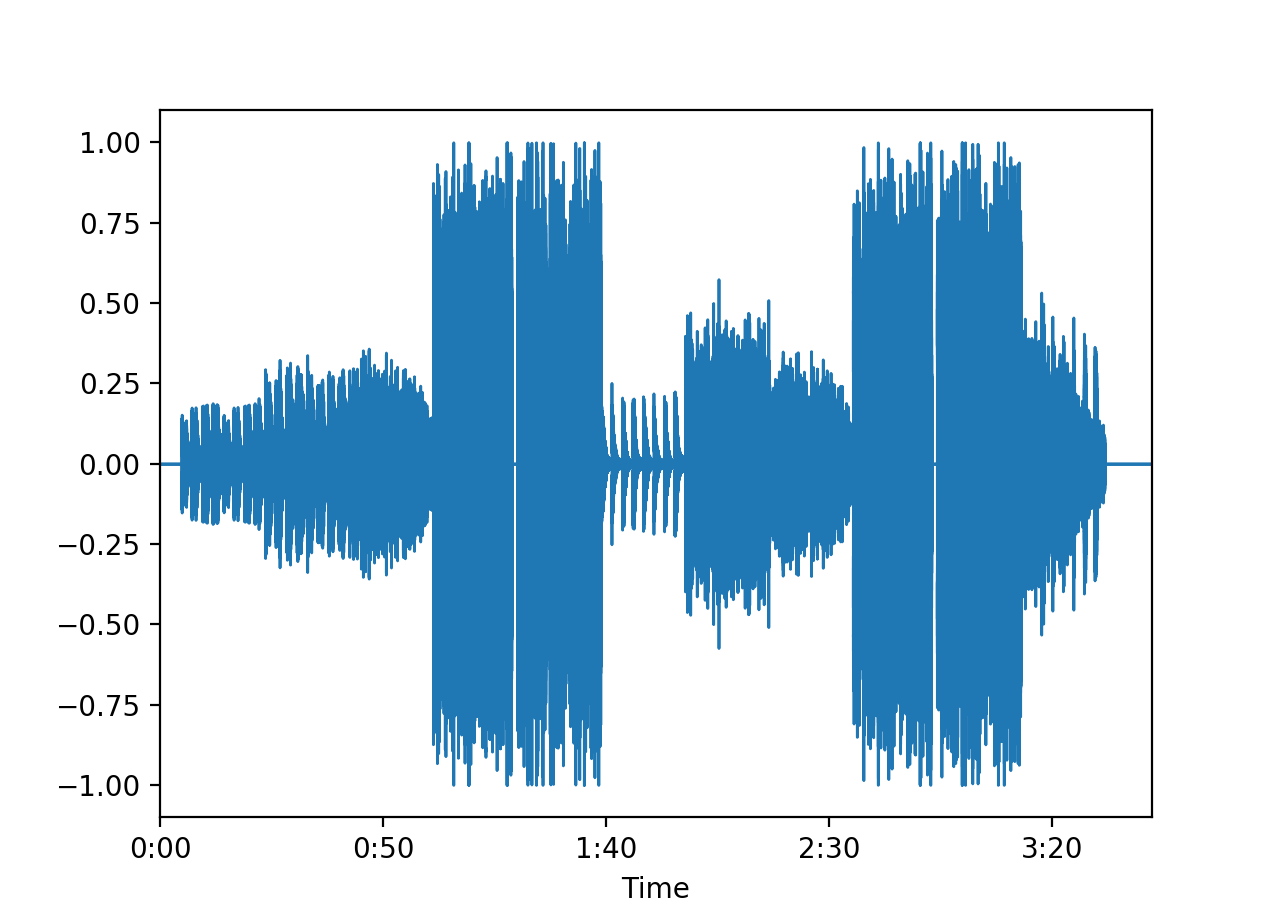分析

音频信号每一位分别对应一个采样点，其位数等于“采样率*音频时长（以秒为单位）”，记录了每个样本上音频的振幅信息

缩放音频信号

原理

音频的响度由音频信号每个样本的数值绝对值大小决定，因此对音频信号作代数乘法运算，可以增减音频的响度

numpy数组提供的代数乘法功能可以用于增减音频响度，并利用librosa库将音频向量写回文件

y=2*y	#增加一倍振幅
y=0.5*y	#减小振幅为原来一半
y=-y	#翻转振幅
y=10*y	#大幅增加振幅
librosa.output.write_wav(dir,y,sr)	#将音频向量写回文件


1
2
3
4
5
结果

四种操作结果的波形分别如图所示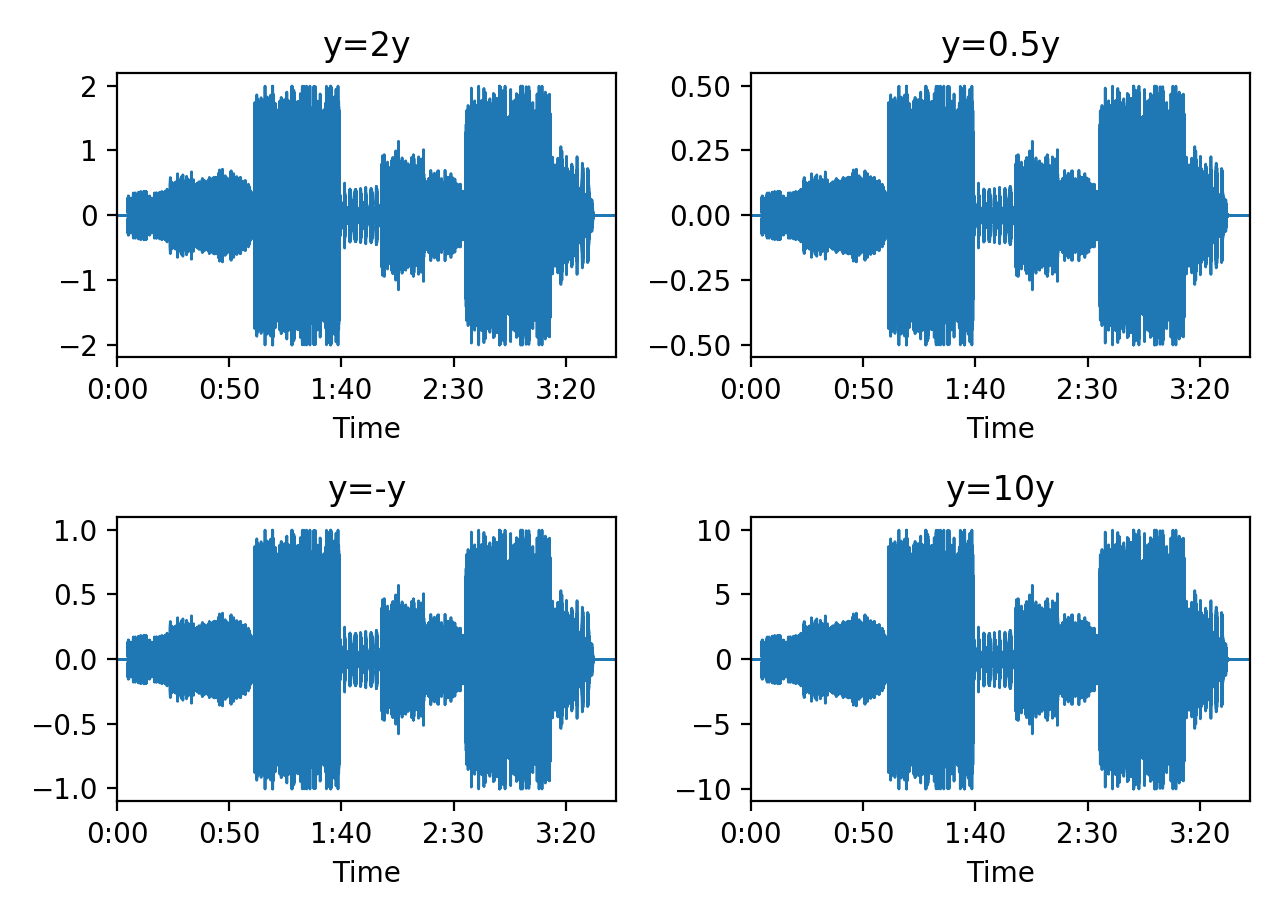y=2*y时，得到的音频响度略大于原音频
y=0.5*y时，得到的音频响度略小于原音频
y=-y时，得到的音频响度与原音频一致
y=10*y时，得到的音频响度远大于原来音频
分析

音频信号每个样本的数值反应了振动离开平衡点的距离，即振幅，振幅越大，声音具有的能量越大，声音听起来响度就越大
对样本数值进行倍增，若倍增系数绝对值大于1则声音响度增加，若倍增系数绝对值小于1则声音响度下降
由于振动离开平衡点的距离是一个绝对值，因此倍增系数为负数时效果与倍增系数是其相反数时一致
线性组合和混音

原理

对多个音频信号进行线性运算y = a 1 x 1 + a 2 x 2 + . . . + a k x k y=a_1x_1+a_2x_2+...+a_kx_ky=a1​x1​+a2​x2​+...+ak​xk​可以实现混音

此时每个音频信号x k x_kxk​称为音轨

混合后的结果y称为混合

每个系数a k a_kak​是音轨在混合中的权重

numpy数组提供的线性运算功能可以用于实现混音

再尝试人声与伴奏的混合，取一段人声轨和一段伴奏轨，分别赋予权重(0.25,0.75)、(0.5,0.5)、(0.4,0.6)进行线性混合

y=0.25*x1+0.75*x2
y=0.5*x1+0.5*x2
y=0.6*x1+0.4*x2


1
2
3
结果

波形图如下所示：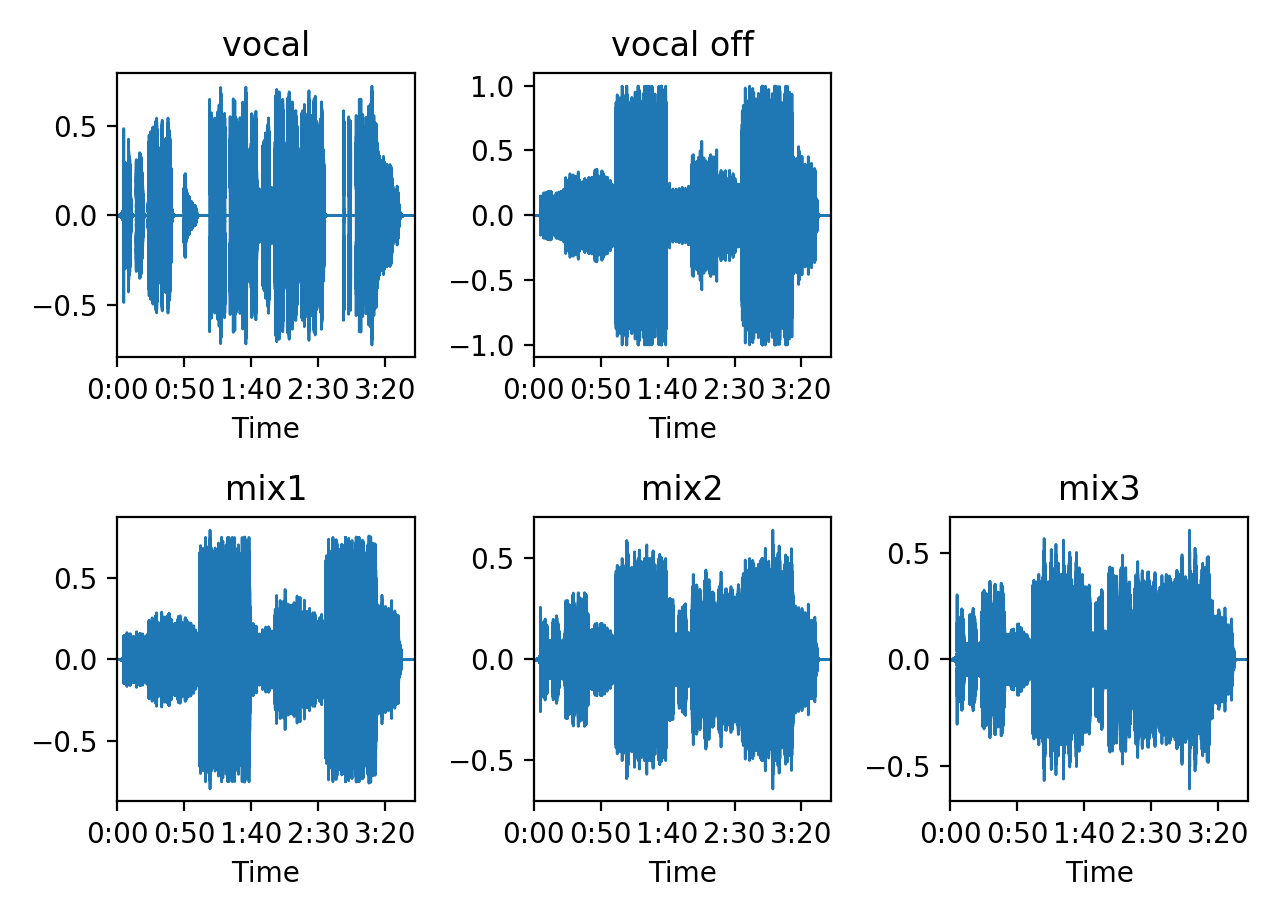得到的音频为在伴奏上加上人声的效果

分析

音频是线性相加，得到的波形图中无法直观分出人声和伴奏，但由于人声和伴奏中各频率谐波分量与伴奏中不同，线性相加在频域被分配到了各频率谐波上，所以可以在频谱图上清晰区分出来

为了得到足够清晰的混音音频，需要不断更改每个混音音轨的权重以得到最适合的混合模式

拓展

虽然人声和伴奏中谐波分量基本不同，但相同频率上的相加也容易导致撞频的发生，使得混合的人声和伴奏难以区分，因此对于双声道音频，我们常常采用偏置的方法，为两个声道中人声和伴奏设不同的权重（通常一个声道人声大，一个声道伴奏声大）

形状为(2,n)的numpy数组可以用于容纳双声道音频信号。两个声道分别取权重(0.4,0.6)、(0.6,0.4)进行混合，波形如下：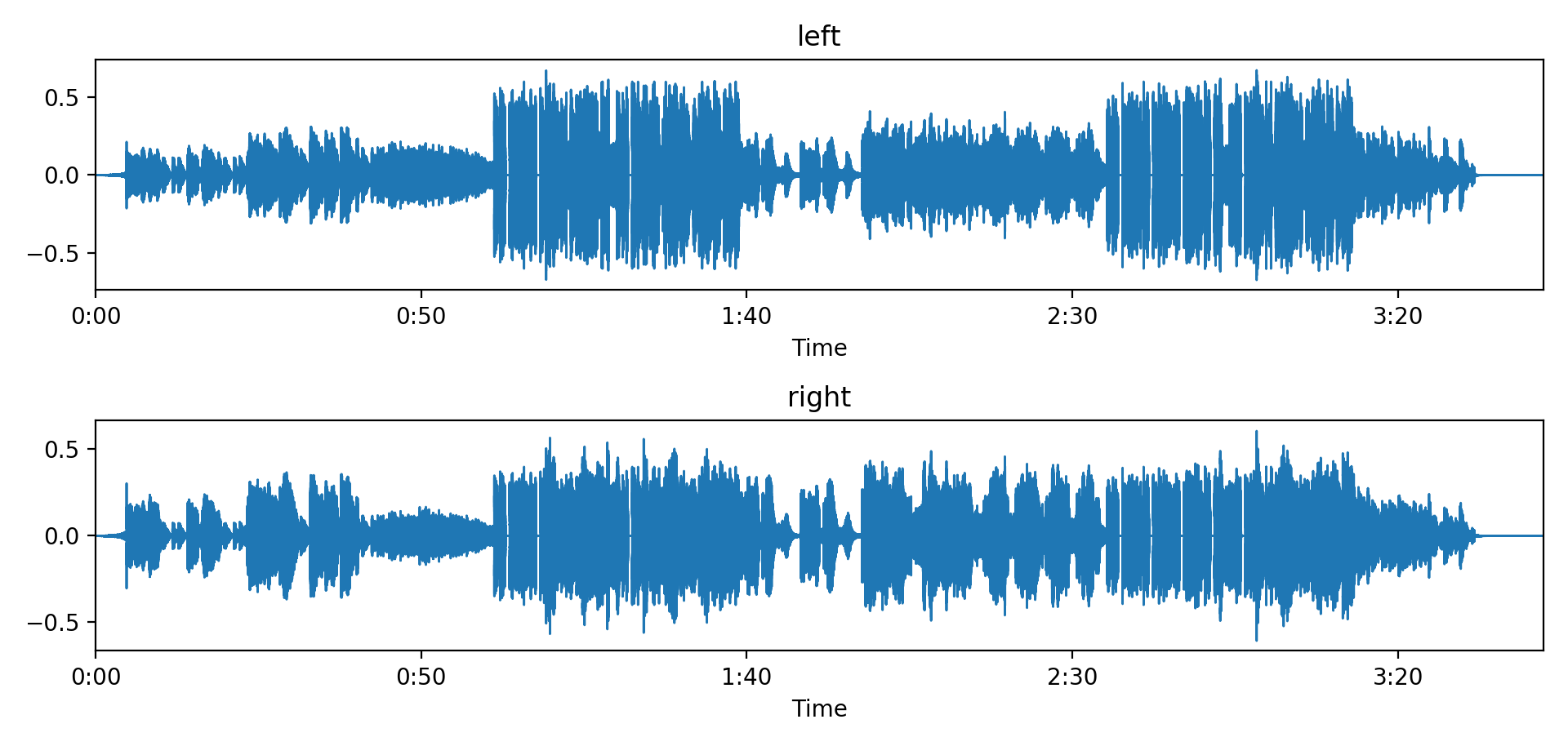乐音

对于声音信号p(t)，若满足p ( t + T ) ≈ p ( t ) p(t+T)\approx p(t)p(t+T)≈p(t)，其中周期T取值在0.0005秒到0.01秒之间，则p被视为乐音
周期长短（决定频率并）决定音高
每个周期内波形的特点决定了音色
乐音的能量决定了响度，即声压
音高

原理

f = 440 H z f=440Hzf=440Hz是中央A
一个八度在频率上翻了一倍
十二平均律中，每个半音在频率上翻了2 1 / 12 2^{1/12}21/12倍
每个半音的距离就是黑白键上两个键之间的距离，从do到升do，各白键之间的差音为全全半全全全半，而每个全音中的半音被分到了黑键上
任意两个音之间的距离称为音程，音程的单位是度，相同单音之间音程为1度，之后每差一个音级增加1度
实验步骤：

设置基频f为440Hz，用numpy以440Hz生成正弦函数
每隔1/44100s设置一个采样点，每间隔1s生成1s的440Hz正弦波
在这基础上，第n次生成时再生成1s频率为440 ⋅ 2 ( n − 1 ) / 12 H z 440·2^{(n-1)/12}Hz440⋅2(n−1)/12Hz的正弦波，与原正弦波线性相加进行和弦，从而模拟小二度到纯八度的所有音程
x=np.empty((0,))	#生成空数组
for i in range(0,13):
x1=np.linspace(0,1, num=44100, endpoint=True, dtype=float)	#生成采样点
x1=5*np.sin(2*np.pi*440*np.power(2,i/12)*x1)+5*np.sin(2*np.pi*440*x1)	#生成波形与和弦
x2=np.zeros((44100,))	#留空部分
x=np.concatenate((x,x1,x2),axis=0)	#连接数组


1
2
3
4
5
6
结果

生成的26s音频中有13段不同音程的和弦，有的和弦听起来和谐，有的和弦听起来不和谐

分析

小二度音程（相差一个半音）、大七度音程（相差五个全音一个半音）是极不协和音程
大二度音程（相差一个全音）、小七度音程（相差五个全音）、三全音（三个全音）是不协和音程
小三度音程（相差一个全音一个半音）、大三度音程（相差两个全音）、小六度音程（相差四个全音）、大六度音程（相差四个全音一个半音）是不完全协和音程
纯四度音程（相差两个全音一个半音）、纯五度音程（相差三个全音一个半音）是完全协和音程
纯八度音程（相差六个全音）是极完全协和音程
音色

对于周期性信号p ( t ) = ∑ k = 1 K ( a k c o s ( 2 π f k t ) + b k s i n ( 2 π f k t ) ) p(t)=\displaystyle \sum_{k=1}^K (a_kcos(2\pi fkt)+b_ksin(2\pi fkt))p(t)=k=1∑K​(ak​cos(2πfkt)+bk​sin(2πfkt))，每个分解信号为谐波或泛音

f为频率

a与b为谐波系数

在K足够大时，任意周期性信号都可以通过积分变换为这种形式

谐波振幅的配比决定了音色，对于每个频率由k决定的谐波，其谐波振幅为c k = a k 2 + b k 2 c_k=\sqrt{a_k^2+b_k^2}ck​=ak2​+bk2​​，因此谐波振幅可以组成一个长度为k的向量c = ( 0.3 , 0.4 , . . . ) c=(0.3,0.4,...)c=(0.3,0.4,...)，足够多的谐波以不同振幅数值混合可以组成不同音色

在220-11000Hz之间分别生成1、5、10、20、50个频段的正弦、余弦信号，随机生成谐波系数

结果

五种情况下混合波形图如下所示：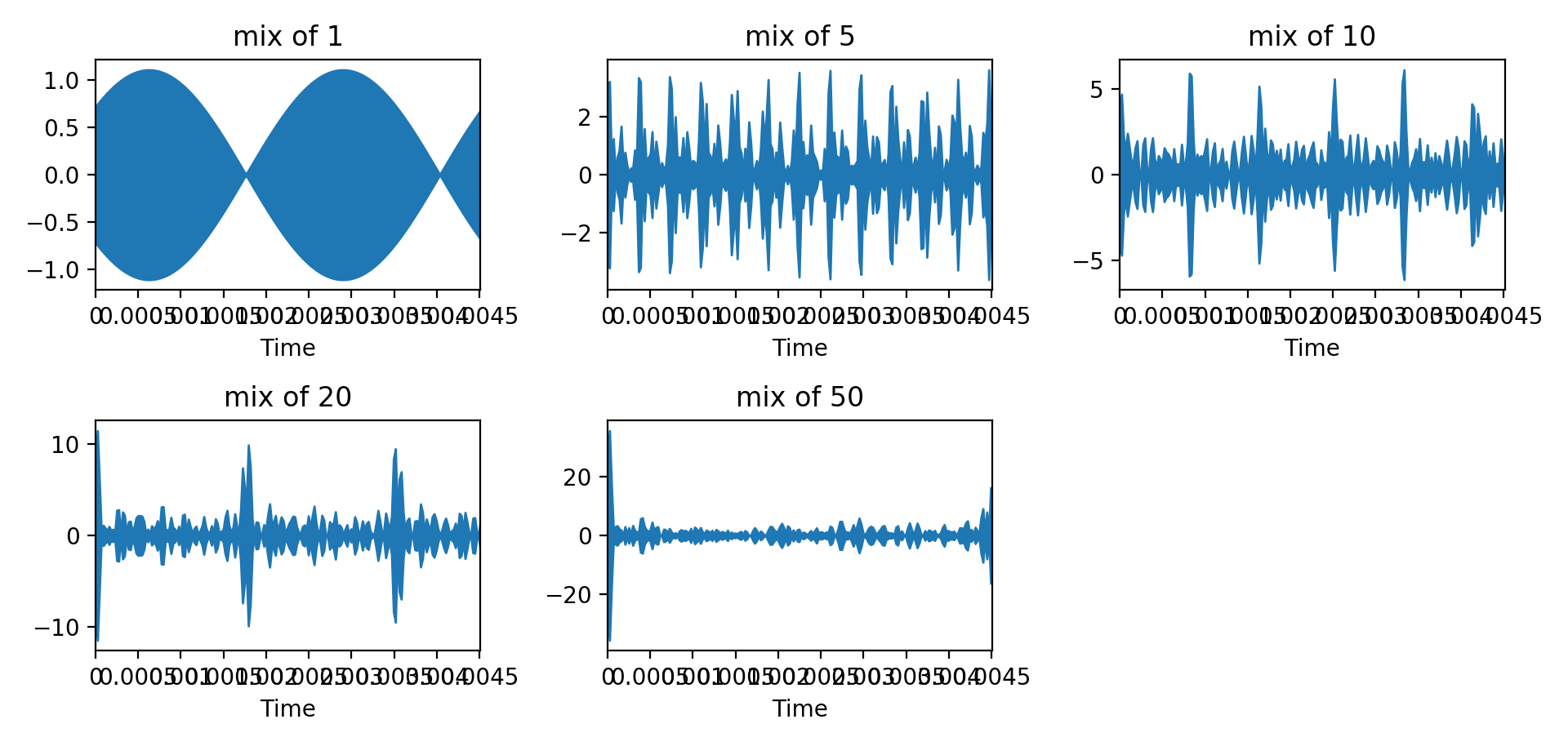得到一段每隔1s播放1s不同音色音频的音频文件

分析

使用不同足够多的谐波以不同谐波系数混合可以组成不同音色音频
现实中已知乐器也可以利用频谱分析提取音色特征，供计算机再现
利用谐波原理可以制作各种各样的电子合成器，供作曲家使用

展开全文• 采样之后，生成音频时间序列 y，其中 len(y) = T * sr = 2 × 24000 = 48000，y.shape = (48000, )，sr=24000。 import librosa # 读取音频 y, sr = librosa.load(path='path.wav', sr=24000) print(len(y), y....


对于时长 T=2s 的音频信号 path.wav，设置采样率为 sr=24000。

采样之后，生成音频时间序列 y，其中 len(y) = T * sr = 2 × 24000 = 48000，y.shape = (48000, )，sr=24000。

import librosa
# 读取音频
print(len(y), y.shape, sr)


设置：

帧移：frame_shift = 0.0125s，帧长：frame_length = 0.05s。（使用帧长 frame_length=0.05s 的窗口对 T=2s 的音频信号进行处理，帧移 frame_shift=0.0125s，则生成的帧数为：2 / 0.0125 = 160(帧) ）

使用sr=24000的采样率，对序列处理：
帧移：hop_length = frame_shift * sr = 0.0125 × 24000 = 300，
window()加窗，窗长：win_length = frame_length * sr = 0.05 × 24000 = 1200。

对音频时间序列 y 进行分帧处理，加窗-帧移之后，得到的帧数 t = len(y) / hop_length + 1 = 48000 / 300 + 1 = 161。目前还不清楚为什么程序处理会多一帧，后续补充。

# 短时傅里叶变换
stft_matrix = librosa.stft(y=y, n_fft=2048, hop_length=300, win_length=1200)
print(stft_matrix.shape)     # shape=(1 + nfft/2 , t), 其中 t=len(y)/hop_length + 1
"""
结果：(1025, 161)
"""



展开全文• 时间：2017-05-06 11:20:47前言本文主要记录librosa工具包的使用，librosa音频、乐音信号的分析中经常用到，是python的一个工具包，这里主要记录它的相关内容以及安装步骤，用的是python3.5以及win8.1环境。...
• 向量x∈RNx\in R^Nx∈RN表示时间区间上的音频信号，xix_ixi​表示t=hit=h_it=hi​时的声压xi=αp(hi),i=1,...,Nx_i=\alpha p(h_i),i=1,...,Nxi​=αp(hi​),i=1,...,N 每个xix_ixi​称为样本 h（>0）为采样时间 1...python 算法
• 作者：桂。... 本文主要记录librosa工具包的使用，librosa音频、乐音信号的分析中经常用到，是python的一个工具包，这里主要记录它的相关内容以及安装步骤，用的是python3.5以及win8.1环境。 ...
• 简单上手-加载音频librosa.load 音符识别的小栗子，可以识别音乐中的音符的起始时间 # Beat tracking example，导入函数库 import librosa # 1. Get the file path to an included audio example audio_path = 'D://...
• librosa.feature.mfcc参数介绍 librosa.feature.mfcc(y=None, sr=22050, S=None, n_mfcc=20, dct_type=2, norm='ortho', **kwargs) ...音频时间序列 sr:number > 0 [scalar] y的采样率 S:...
• ## librosa入门学习

千次阅读 2018-12-12 15:52:31python
• librosa.feature.mfcc参数介绍 librosa.feature.mfcc(y=None, sr=22050, S=None, n_mfcc=20, dct_type=2, norm=‘ortho’, **kwargs) ...音频时间序列 sr:number > 0 [scalar] y的采样率 S:np.ndar...
• #调用音频处理库加画图 import librosa from librosa import display import numpy as np import matplotlib.pyplot as plt #音频播放 import pyaudio ...#加载音频，x时间序列 sr采样率 x , sr = librosa.loadpython
• ## librosa.effects.split

千次阅读 2019-12-04 21:18:30
librosa.core.load librosa.core.load(path, sr=22050, mono=True, offset=0.0, ...y：np.ndarray [shape =（n，）或（2，n）] 音频时间序列 sr：number> 0 [标量] y的采样率 y, sr = librosa(filena...
• 使用Librosa音频处理库和openSMILE工具包，进行简单的声学特征提取，包括韵律学特征(持续时间、短时能量、过零率、基频等)、基于谱的相关特征(MFCC特征)和声音质量特征(共振峰、频率微扰、振幅微扰)。如果您觉得有.....
• 本文要实现的目标为： 假如：1个音频的总帧数=170880,采样率sr=48000,持续秒数=3.560000 假设音频数据为y： y = [-0.00856018 -0.00930786 -0.00827026...要转化成的时间为times： times = [ 0.00000000e+00 2.0833...
• 时间序列（time series）：一种典型的音频信号，用y表示，代表一个一维的浮点数组。y[t]对应数组中位于t点的幅度值。 采样率（sampling rate）：时间序列每秒的采样数（值为正整数），由整数变量sr表示。 帧...信号处理 机器学习 python
• 包括对读取的音频数据的dft（离散傅里叶变换）、idft，stft（短时傅里叶变换）、istft，ssa（奇异谱分析），分帧和复原。 # coding=utf-8 """ 对音频数据的几种数据转换...import librosa import scipy.io.wavf...
• **功能实现：**读取音频文件的数据，取其中一部分数据，生成幅度谱，相位谱。 import numpy as np import pylab as plt import librosa from math import * import wave import time time_start=time.time() def ...python 蝶形运算 fft
• 频谱图是音频的一个重要特征，在深度学习算法中也经常用到，是通过视觉表示频谱的频率、声音或其他信号，因为它们随时间变化。频谱图有时被称为超声波仪，声纹或语音图。二维矩阵中一般第一维是频率，第二维是时间。...机器学习 深度学习
• 对于音频，变化的量是气压。 我们如何以数字方式捕获此信息？ 我们可以随时间采集气压样本。 我们采样数据的速率可以变化，但是最常见的是44.1kHz，即每秒44,100个采样。 我们捕获的是信号的波形，可以使用计算机...信号处理 机器学习
• import matplotlib.pyplot as pltimport librosa.displayplt.figure(figsize = (14,5))librosa.display.waveplot(x, sr = sr)In#谱图：谱图是通过视觉表示频谱的频率，声音或其它信号，因为它随时间变化。...
• Python 音频信号处理库 librosa–入门 1，音频处理 filename = librosa.example('...以时间序列的 y形式加载和解码音频，以一维NumPy浮点数组表示y。 可变SR包含采样速率的 y，即，每秒音频的样本的数目。默认情况
• ## 声学特征提取-python

千次阅读 热门讨论 2020-05-11 22:26:11
使用Librosa音频处理库和openSMILE工具包，进行简单的声学特征提取，包括韵律学特征（持续时间、短时能量、过零率、基频等）、基于谱的相关特征（MFCC特征）和声音质量特征（共振峰、频率微扰、振幅微扰）。...python 语音识别 可视化python samplerate
• 音频信号进行采样采样是指将连续时间信号转换为离散时间信号的过程。 采样频率/采样率是每秒的平均采样数。 f_S = 1 / T我们还将使用频谱图表示音频信号。 频谱图是一种直观地表示特定音频信号的波形中可用的不同...
• librosa库的几个用法 Parameters： path要输入文件的路径 sr设置采样率 ...offset 浮点型，从这个时间开始读取音频 duration 浮点型 音频的持续时间 res_type 字符型用途似乎是关于采样质量的 ...
• GenVIdeo 一种基于python编写的视频自动生成程序 一，想要设计的功能： 1，文字转视频任意输入或利用爬虫...二，目前需要的python依赖： moviepy，pymysql，json，解霸，urllib，librosa，uuid，数据时间，百度Aip moPython